# Beta

In this article, you'll learn about beta, how to use it to evaluate the relationship between a symphony and its benchmark, and how it's calculated in Composer.

What is beta?

Beta is a measure of how sensitive a symphony is to change in a selected benchmark. It measures the typical change in the symphony relative to a given change in the benchmark. The further away the beta is from zero, in either the positive or negative direction, the more sensitive a symphony is to change in the benchmark.

How does it work? Linear regression is used to model the relationship between the performance of the benchmark and that of the symphony over a given time period. The daily percent returns of the benchmark are regressed on the daily percent returns of the symphony. Beta is the slope of the regression line.

A beta of 1 means that for every 1% increase in the returns of the benchmark, we’d expect a 1% increase in the returns of the symphony. A beta closer to zero means that the symphony is less sensitive to changes in the benchmark. For example, a beta of .5 means that for every 1% increase in the returns of the benchmark, we’d expect a .5% increase in the returns of the symphony. A beta greater than 1 means that the symphony is more sensitive to changes in the benchmark. For example, a beta of 2 means that for every 1% increase in the returns of the benchmark, we’d expect a 2% increase in the returns of the symphony.

A negative beta indicates that increases in the performance of a benchmark correspond to decreases in the performance of the symphony. A beta of -1 means that for every 1% increase in the returns of the benchmark, we’d expect a corresponding 1% decrease in the returns of the symphony. A beta lower than -1 means that the symphony is more sensitive to changes in the benchmark. For example, a beta of -2 means that for every 1% increase in the returns of the benchmark, we’d expect a 2% decrease in the returns of the symphony.

It’s important to consider the beta together with the R2 to evaluate the relationship between a symphony and a benchmark. If the R2 is sufficiently low, meaning that little variation in the symphony’s performance can be explained by variation in the performance of the benchmark, this indicates that the beta is unreliable.

Where will you see beta in Composer?

You’ll see beta in backtest results in a section on measures of correlation.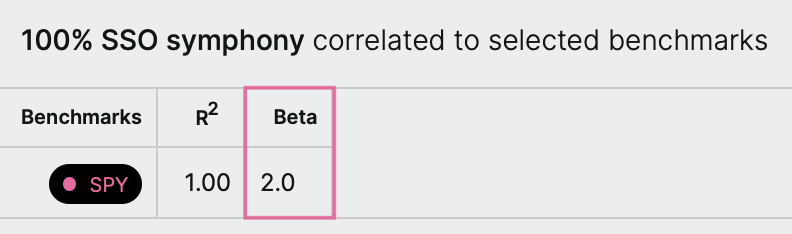Examples

Let’s look at a few examples.

First, let’s take a look at a symphony made up of 100% \$SPY with a benchmark of \$SPY. As we’d expect, the beta is 1, as the symphony is perfectly sensitive to changes in the benchmark. A 1% increase in the returns of the benchmark corresponds to a 1% increase in the returns of the symphony.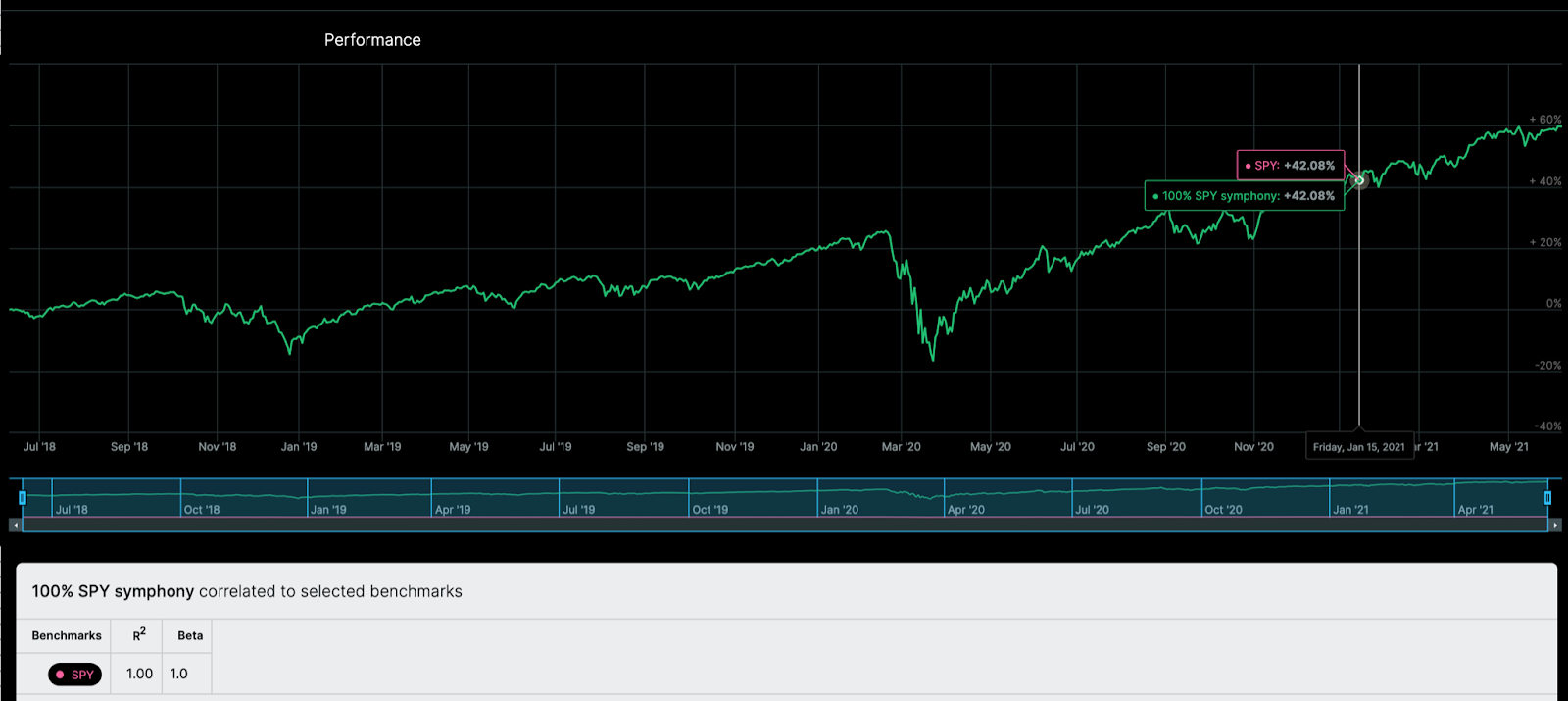Next, let’s take a look at a symphony made up of \$SSO, a leveraged ETF that seeks daily returns that correspond to two times the daily performance of the S&P 500. Here, the beta is 2, as the symphony is twice as sensitive to changes in the benchmark. A 1% increase in the returns of the benchmark (SPY) corresponds to a 2% increase in the returns of the symphony (SSO).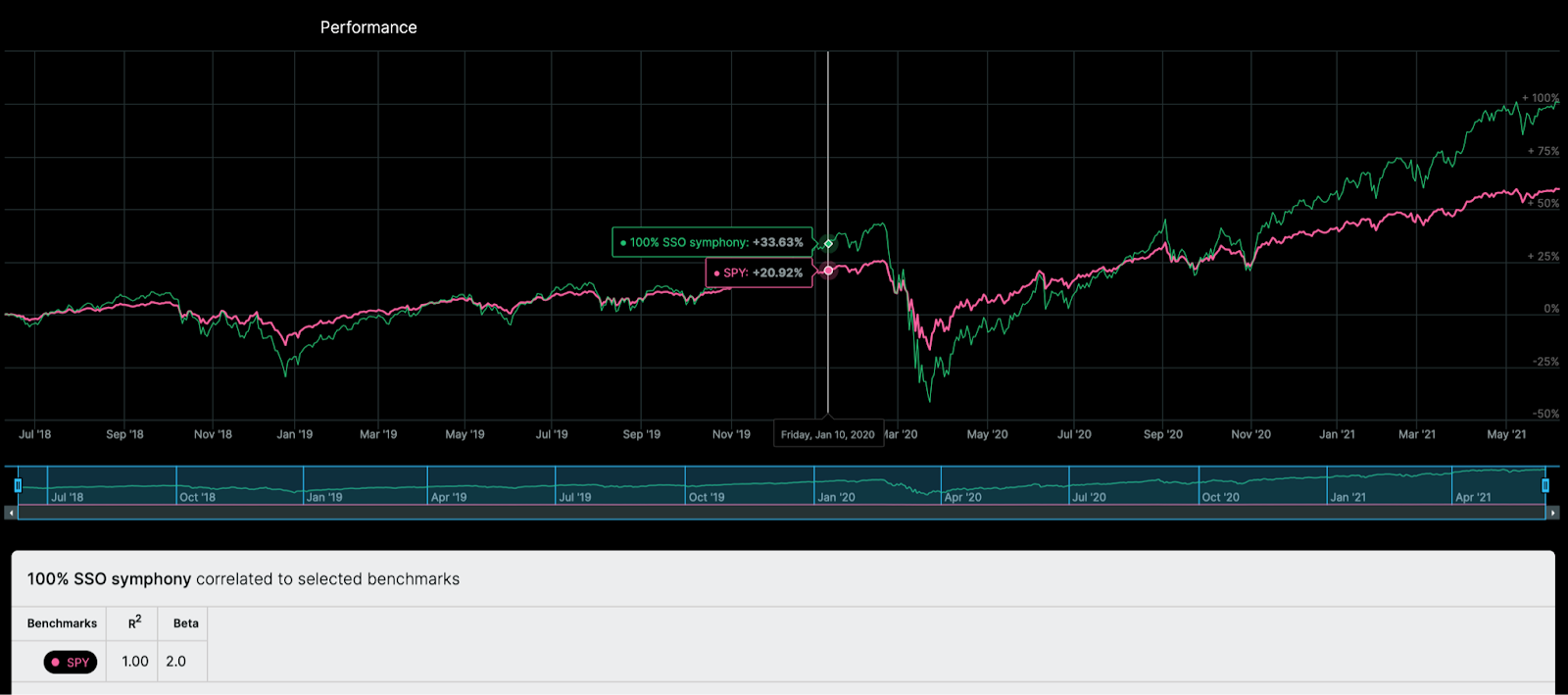Finally, let’s take a look at a symphony made up of 100% \$SPY with a benchmark of \$SSO. In this case, the symphony is less sensitive to changes in the benchmark, and the resulting beta is .5. A 1% increase in the returns of the benchmark (SSO) corresponds to a .5% increase in the returns of the symphony (SPY).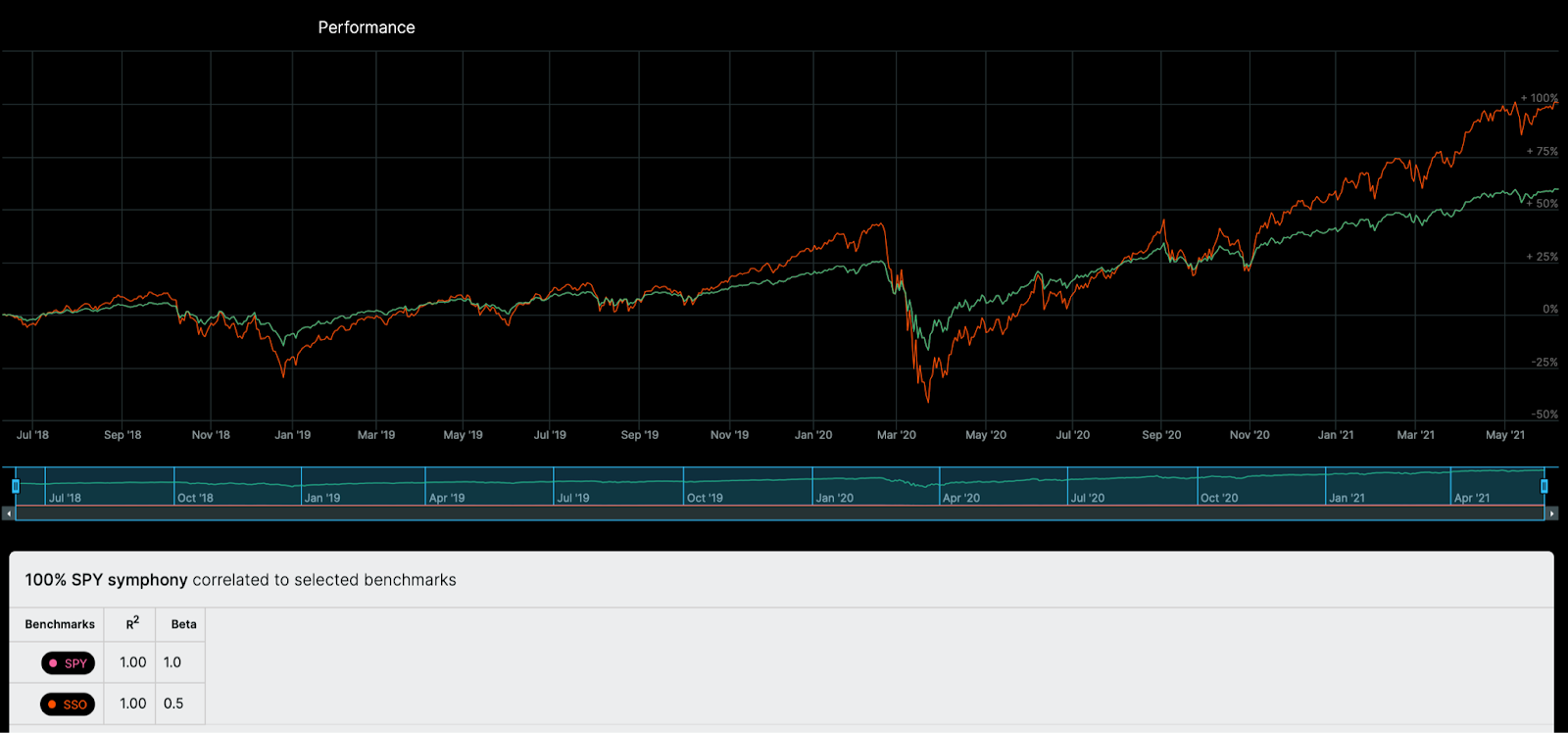What is the formula?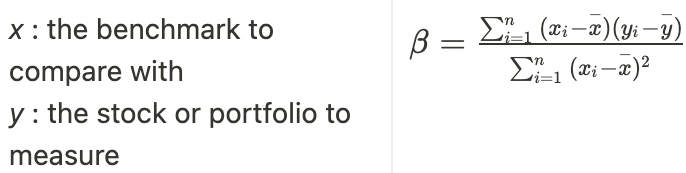What is the step-by-step calculation?

Let's go through the calculation of the beta step-by-step for a symphony backtest. Here's how we do it:

• Start with the daily returns for the symphony and the benchmark over the selected time period.
• Compute a linear regression that predicts symphony daily returns from benchmark daily returns.
• Display the beta from the linear regression equation.